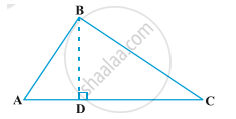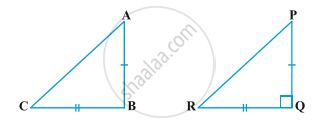Share

# Pythagoras Theorem

#### theorem

Theorem1: In a right triangle, the square of the hypotenuse is equal to the sum of the squares of the other two sides.Given: A right triangle ABC right angled at B.
Construction: Draw BD ⊥ AC
To prove: "AC"^2= "AB"^2+"BC"^2
∠A= ∠A (common)
∠BDA= ∠ABC= 90°

So, "BA"/"AC" = "AD"/"BA" (Corresponding parts of Congruent triangles)

"BA"^2 = "AC"xx "AD" .....eq1
Again, In ∆BCD and ∆BAC
∠C= ∠C (common)
and ∠BDC= ∠ABC= 90°
Therefore, ∆BCD ∼ ∆ACB (Angle-Angle similarity)

"BC"/"AC" = "CD"/"BC" (Corresponding parts of Congruent triangles)

"BC"^2= "AC"xx "CD" ......eq2
"BA"^2 + "BC"^2 = ("AC"xx "AD")+ ("AC"xx "CD")
= "AC" ("AD"+"CD")
= "AC" xx "AC"
"BA"^2 + "BC"^2 = "AC"^2
Hence proved.

Theorem2:  In a triangle, if square of one side is equal to the sum of the squares of the other two sides, then the angle opposite the first side is a right angle.Given: A triangle ABC such that "AC"^2= "AB"^2+"BC"^2
To prove: ∠B= 90°
Construction: Draw a triangle PQR such that PQ=AB, QR=BC and ∠Q=90°
Proof: In ∆PQR,
"PR"^2= "PQ"^2+"QR"^2 (Pythagoras theorem)
"PR"^2= "AB"^2 + "BC"^2 (by construction) .....eq1
"AC"^2= "AB"^2 + "BC"^2 (Given) .....eq2
From eq1 and eq2
Therefore, "PR"^2= "AC"^2
PR= AC
Now, In ∆ABC and ∆PQR,
AB= PQ (by construction)
BC= QR (by construction)
and AC= PR (proved above)
Therefore, ∆ABC ≅ ∆PQR (Side-Side-Side rule)
So, ∠B=∠Q= 90°  (Corresponding parts of Congruent triangles)
Therefore, ∠B= 90°
Hence proved.

#### description

• Pythagoras Theorem : In a right-angled triangle, the square on the hypotenuse is equal to the sum of the squares on the remaining two sides.
• In a right-angled triangle, the square on the hypotenuse is equal to the sum of the squares on the remaining two sides.
If you would like to contribute notes or other learning material, please submit them using the button below.

### Shaalaa.com

Triangles part 38 (Proof Pythagoras Theorem) [00:11:12]
S